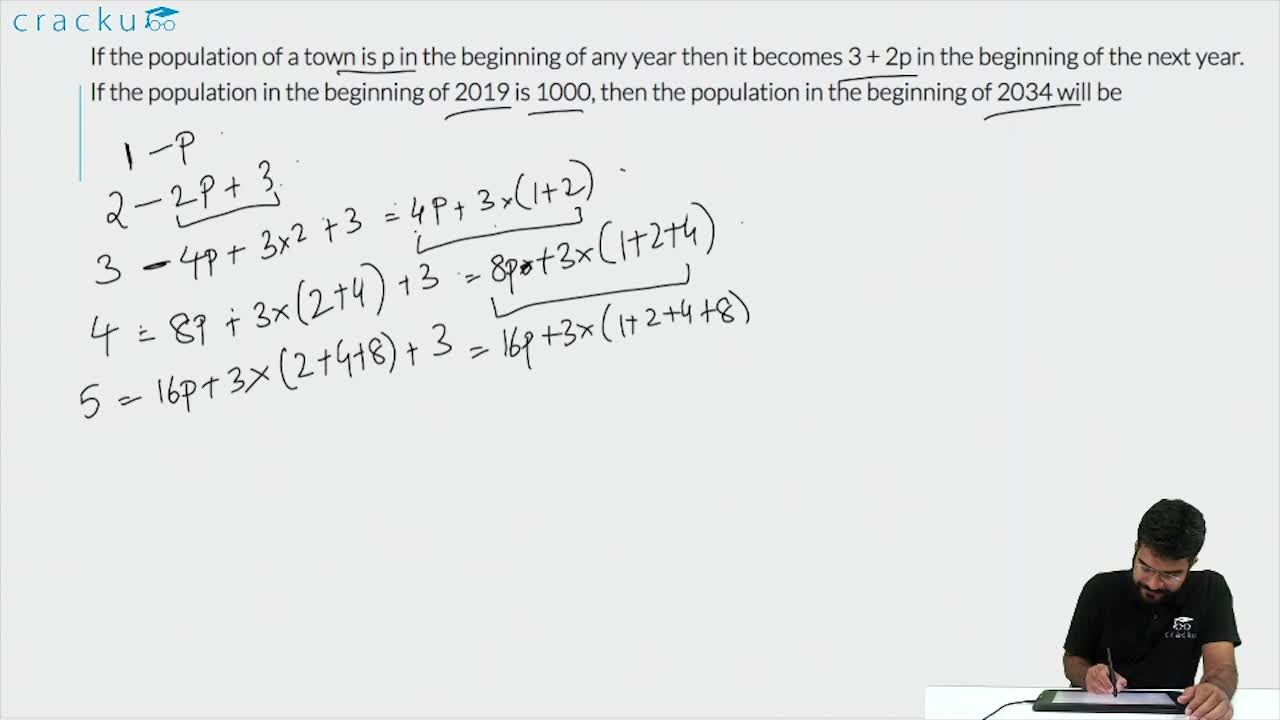Question 75

# If the population of a town is p in the beginning of any year then it becomes 3 + 2p in the beginning of the next year. If the population in the beginning of 2019 is 1000, then the population in the beginning of 2034 will be

Solution

The population of town at the beginning of 1st year = p

The population of town at the beginning of 2nd year = 3+2p

The population of town at the beginning of 3rd year = 2(3+2p)+3 = 2*2p+2*3+3 =4p+3(1+2)

The population of town at the beginning of 4th year = 2(2*2p+2*3+3)+3 = 8p+3(1+2+4)

Similarly population at the beginning of the nth year = $$2^{n-1}$$p+3($$2^{n-1}-1$$) = $$2^{n-1}\left(p+3\right)$$-3

The population in the beginning of 2019 is 1000, then the population in the beginning of 2034 will be $$(2^{2034-2019})\left(1000+3\right)$$-3 = $$2^{15}\left(1003\right)$$-3

### View Video Solution